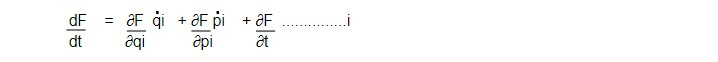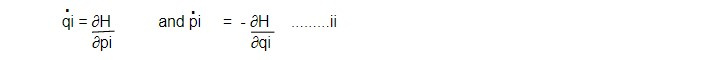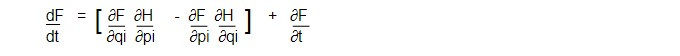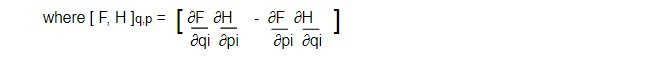# Poisson Bracket

ELI5: Imagine you have a big toy box filled with different toys like cars, dolls, action figures, etc. Each toy represents a different thing, like the position and speed of a car, or the temperature and humidity of the air. The Poisson Bracket is like a tool that helps us see how all these different toys are related to each other and how they change over time.

So let's say you have a toy car and a toy airplane in the box. You might notice that when the car moves faster, the airplane moves slower. Or maybe when the temperature goes up, the humidity goes down. The Poisson Bracket is like a special tool that helps us see these connections and understand how the different toys in the box are related to each other and how they change over time.

---

The Poisson bracket is a mathematical concept that is related to Hamilton's equations, which are a set of equations used in physics to describe the behavior of systems that have a certain type of symmetry, known as Hamiltonian symmetry.

The Poisson bracket is a way of expressing the relationship between two different quantities, called "functions," in a system that has Hamiltonian symmetry. It is defined as the function that results from the product of the partial derivatives of one function with respect to two other functions.

In Hamilton's equations, the Poisson bracket is used to express the time evolution of the system in a way that reflects the Hamiltonian symmetry. These equations describe how the position and momentum of a particle change over time, and they are widely used in physics, particularly in the study of classical mechanics and quantum mechanics.

In simple terms, Poisson bracket is a way to express the relationship between different variables in a system and Hamilton's equation is a set of equations that describe how these variables change over time.

---

Poisson Bracket is the key component of Hamilton's equations of motion, which controls the temporal development of a Hamiltonian dynamical system. It governs the time evolution of a Hamiltonian dynamical system.

Derivation of Poisson Bracket

If F be a function of canonical variables qi and pi and possibly of time, then we have,From canonical transformation equation, we haveUsing eq ii and i we get= [F,H] qipi + ∂F/∂tis called poisson bracket of function F and H with respect to canonical variables q and p.

Similarly if x and y are the function of canonical variables qi and pi then the poisson bracket of x and y with respect canonical variable qi and pi can be expressed asProperties of Poisson bracket

i. [x,y ] q,p = - [y,x]q,p

ii. [x,x]q,p =0

iii. [x,yz] q,p = [x,y]q,p z + y [x,z]q,p

iv. [x, y+z] q,p = [x,y]q,p + [x,z]q,p

v. [ax+by,z]q,p = a[x,z]q,p + b[y,z]q,p

vi. If C is independent of q and p or both then [x, C]q,p = 0

vii. ∂/∂t [x,y] q,p = [∂x/∂t,y]q,p + [x, ∂y/∂t ]q,t

viii. [x, qi]q,p = -∂x/∂pj

and [x, pj ]q,p = ∂x/∂qj

This note is taken from Classical Mechanics, MSC physics, Nepal.

This note is a part of the Physics Repository.